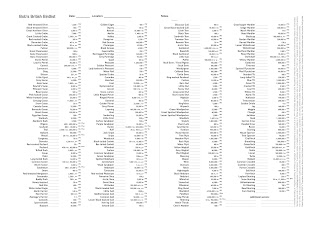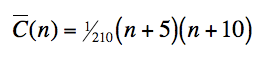## Wednesday, March 11, 2009

### British BirdlistsToday I finished off two neat little bird lists. If you click on either the images below, you can grab yourself a copy.

The first is a straightforward list of all the native birds of Great Britain. If you print it and fold into quarters, you can slip it in your bird guide on a day out and make a note of what you've seen as you go.I've included all species for which, on average, more than 5 pairs breed or more than 50 non-breeding individuals visit each year. There are 247. I've wanted a list like this for ages, to encourage me to record what I see while I'm out. I'm sure there are similar lists out there, but I couldn't find one exactly how I wanted it... so I've done it myself.

The second list consists of the same 247 species of birds, but with a note of how many of each species there are, because sometimes that's a very useful thing to know...The note alongside each bird on the list indicates: the number of breeding pairs; whether they're predominantly resident (r) or migratory (m) breeders; how many individuals are present in winter (w) (or a note such as "w+" if winter numbers are 2-5 times higher than summer; a double ++ implies 5 to 20 times); and a note of how many passage migrants (p) there are, where these are significantly higher than both summer and winter populations. Where no note for winter is given, the number of wintering resident adults is similar to the population in summer – roughly 3x the number of resident breeding pairs – and the number of wintering migrants is zero or extremely low.

Most of the information is simplified from the 2006 report by the Avian Population Estimates Panel (APEP).

(Abbreviated notes are included right-hand side of the sheet.)

Adding up the numbers, it seems that there are around 66 million breeding pairs of birds in Great Britain – still a little more than one pair for every human being.

Long may it stay that way.

## Saturday, February 07, 2009

### Rugbinatorics

The Six Nations Championship started today. I tried to watch the first game, but got distracted by the pretty numbers.

The scoring possibilities at any point in the game are 3 (drop goal or penalty goal, g), 5 (unconverted try, u) or 7 (converted try, c). Some scores (e.g. 4) are not possible at all. Some (e.g. 10 = cg or uu) are possible in more than one way. What the blazes is going on? There must be a formula.

It's best to start by ignoring goals and look at what's possible using tries alone. The table below is the set of scores uniquely possible from tries alone – converted or unconverted. The word uniquely implies that we exclude scores like 35 which could be generated in two distinct ways (u×7 or c×5). There are 35 such uniquely generated scores, and each takes the form 5a+7b, with 0≤a<7 and 0≤b<5.Let's call this set A.

Adding 35 to any of these numbers will give a score that can be made in two ways by tries alone (simply because the 35 can be made in two ways, and the rest can be made in one). Adding 70 will give a score that can be made in three ways... and so on.

To introduce drop goals, we add multiples of 3 to the numbers above. For example, 19 can be scored in 3 ways: it is 10 (in the table) plus 3 goals; or 7 (in the table) plus 4 goals; it's also in the table in its own right (two converted tries and one unconverted) plus no goals.

It's helpful to arrange the 35 scores of set A into modulo 3 subsets – that is, to divide them into those divisible by 3 without remainder, those with remainder 1 and those with remainder 2.This gives us three subsets, which we can call set 0, set 1 and set 2.

Taking our example of how 19 may be score, we see that it is present in set 1, and that there are two smaller numbers in the set. We know that these numbers – 7, 10 and 19 – can be scored by tries alone, and by adding the required number of drop goals, each can be made up to our score of 19. The three ways of scoring 19 are more readily visible using this table.

Thus, for scores less than 35, the number of ways C(n) of reaching a score n is given by the number of members of the set n mod 3 (i.e. set 0, 1 or 2, shown left) which are less than or equal to n.

If n ≥ 35, we must supplement the result from the partial formula above by considering the two ways in which 35 may be scored by tries (u×7 or c×5).

For example a score of 50 (which divides by three with remainder 2) can be made using any of the unique combinations of tries in set 2 (there are 11 of these) together with the required number of drop goals. In addition, we can score 35 by tries – in either of the two ways – plus 15. The above partial formula gives us 3 ways of scoring when n = 15. With two ways of scoring 35 in each case, we can therefore add 6 further ways to reach 50. Together with the 11 found earlier, we have a total of 17 ways of scoring 50 points.

For a general approach, we can proceed as follows. Obtain a starting result using the partial formula above. Then subtract 35, and obtain a second result, and multiply this by 2. If n ≥ 70, subtract a further 35, obtain a third result, and multiply this by 3. Continue in this way until no further 35 can be subtracted, and this is the final result.

The number of ways C(n) of reaching a given score n in Rugby Union is thus:where A{n mod 3, ≤ n} is defined as the number of members of n's modulo 3 subset, 0, 1 or 2, of set A (which is the set of integers 5a+7b: 0≤a<7, 0≤b<5; as tabulated above left) which are ≤n. The sum is from r=0 to the integer quotient of n/35.

Example: a score of n=100...
n/35 is 2 and a bit, so we sum from r=0 to r=2.

r=0: 100 mod 3 = 1; there are 12 members of set 1, all ≤ 100. 1 × 12 = 12.
r=1: 65 mod 3 = 2; there are 11 members of set 2, all ≤ 65. 2 × 11 = 22.
r=2: 30 mod 3 = 0; there are 7 members of set 0 which are ≤ 30. 3 × 7 = 21

The total number of ways of scoring 100 is 55.

It would be straightforward to list all 55 should we wish. For example, there are 21 entries in the third (r=2) part of the total above: three for each member of set 0 up to and including 30. If we chose, say, the second member, which is 12 (corresponding to uc in the notation set up at the beginning), we can pinpoint the three ways of scoring 100 associated with it. The 12 from set A in this case represents scoring 30 by uc g×6 . The remaining 70 may be either u×14 or u×7 c×5 or c×10 – the three combinations represented in the sum. The precise contribution of any member of each set included in the steps of the sum can be identified and listed by this process.

The result follows extraordinarily closely to a quadratic function:Using this approximation for C(n), and rounding to the nearest whole number, gives the correct answer in over 80% of cases, and I've yet to find a value of n for which it is out by more than 1. (For n=100, it gives a neat 55.)

I can see where the 1/210 comes from. Where n is a multiple of 35, summing (r+1) from r=0 to n/35 - 1 gives (n/35 )(n/35 +1)/2, then multiply this by the average full set of A, which is 35/3, and you have your 1/210 coefficient of n². But I can't for the life of me figure out why there's a consistent coefficient of 1/14 for n. So there's a puzzle for you.

So now you see how hours of fun may be had at a rugby union match without any need to understand of the rules of the game. One might be tempted to conclude that the game hardly need be played at all. But if it is, then a team being 4 points behind towards the end of a match would do well to be aware that, by my calculations, were they to score one more try, they'd be winning.